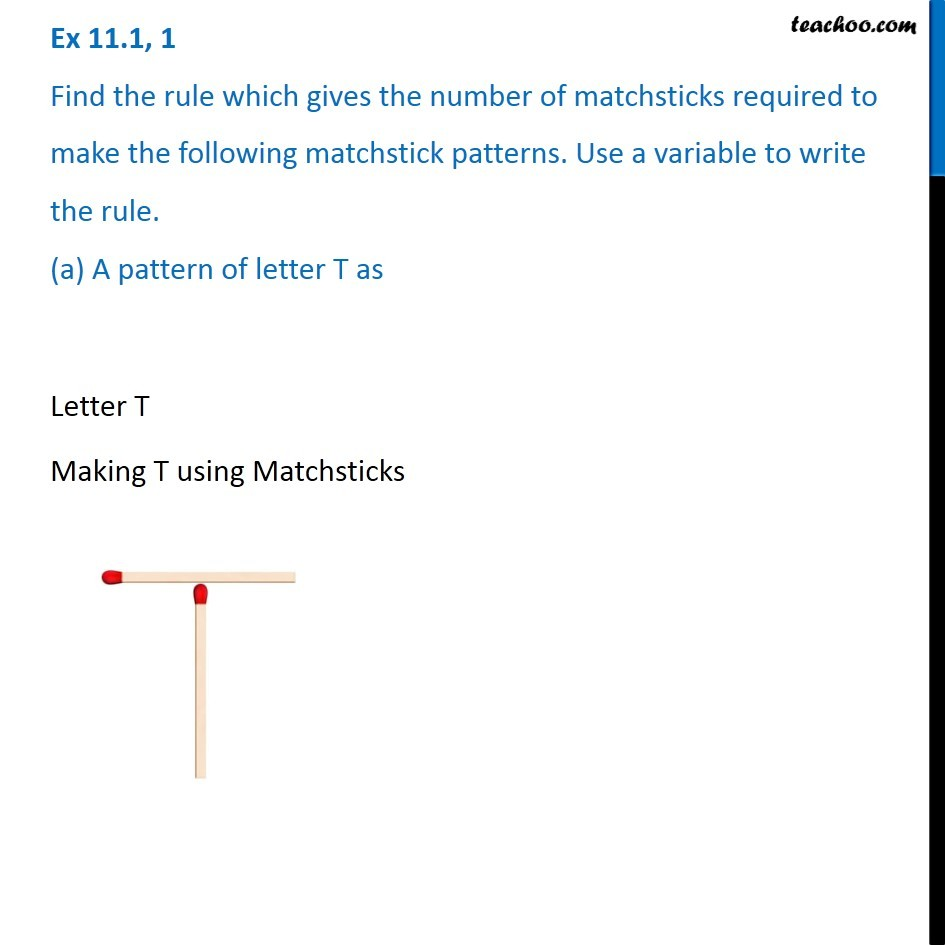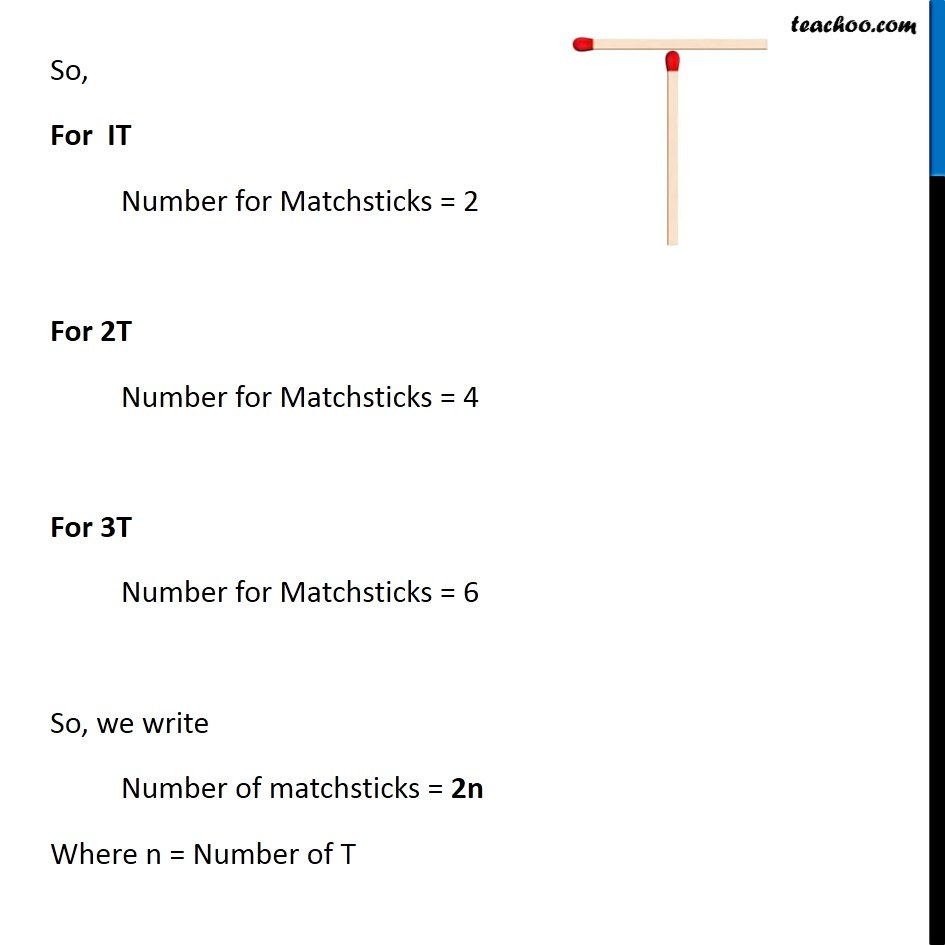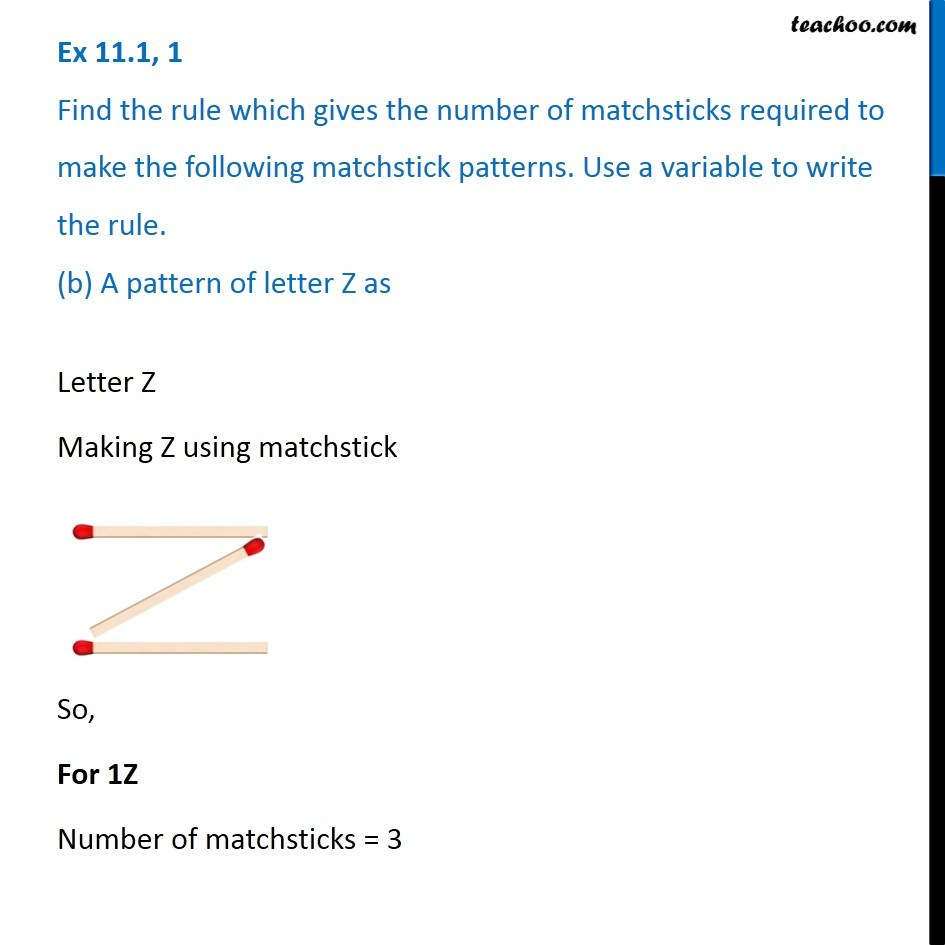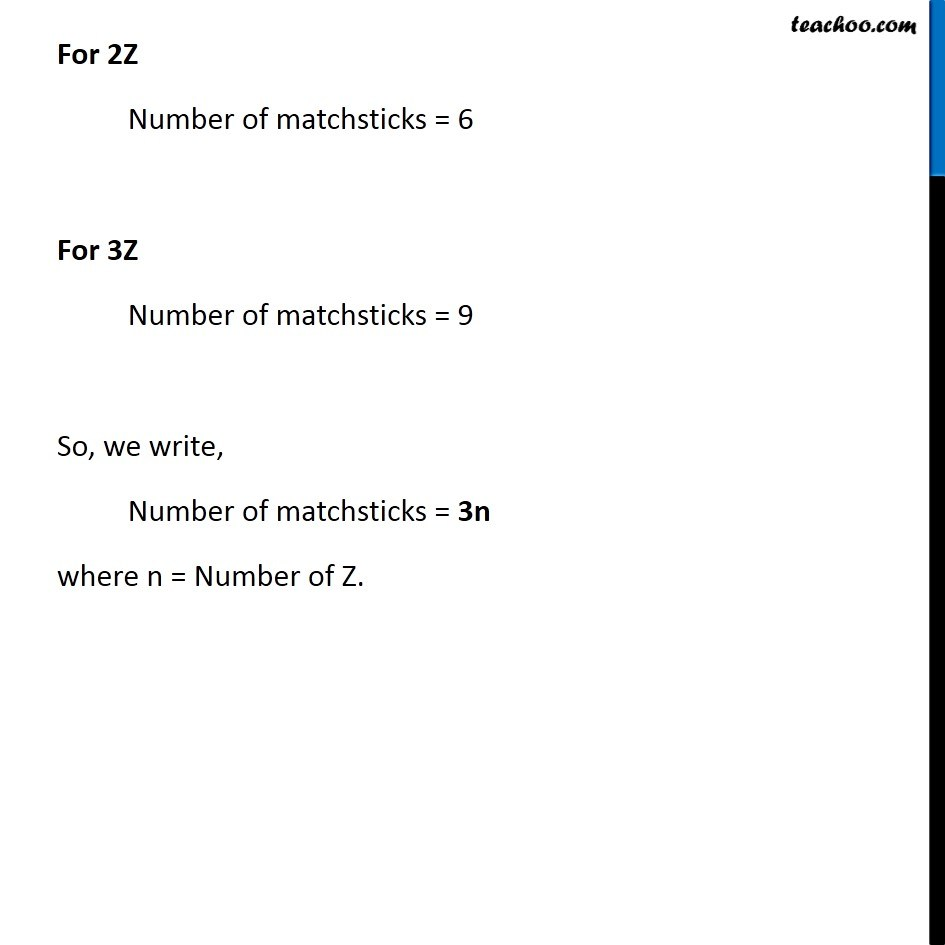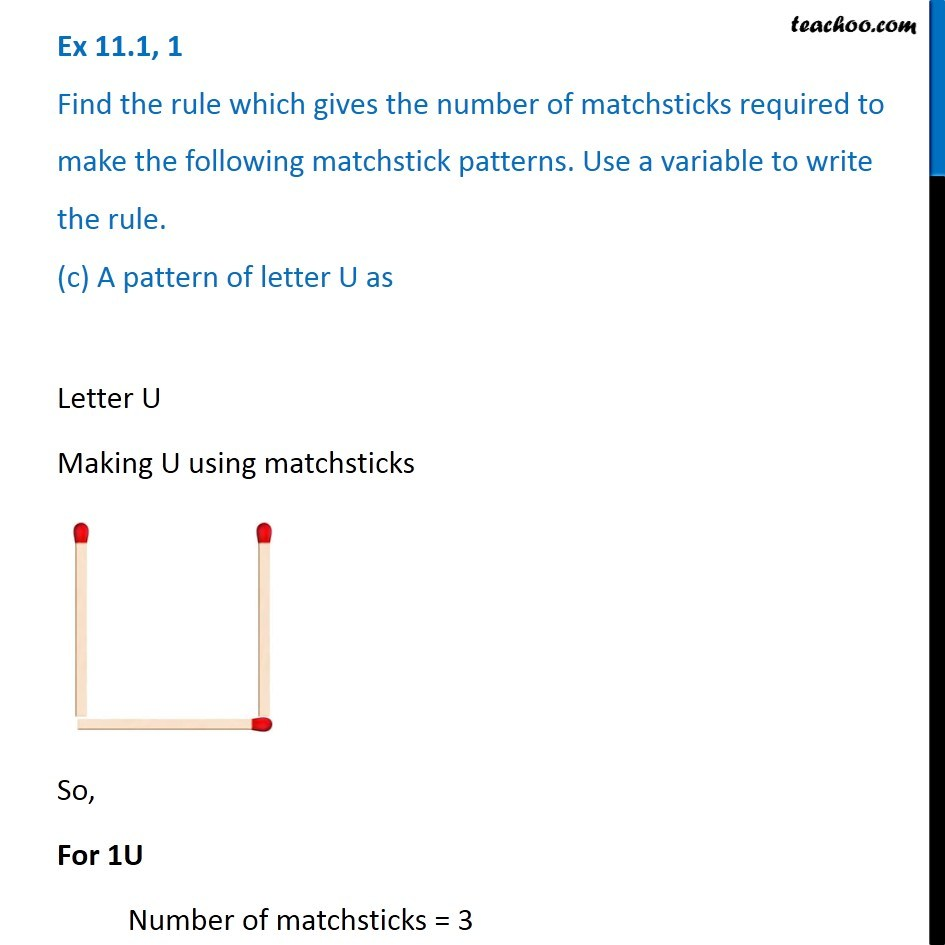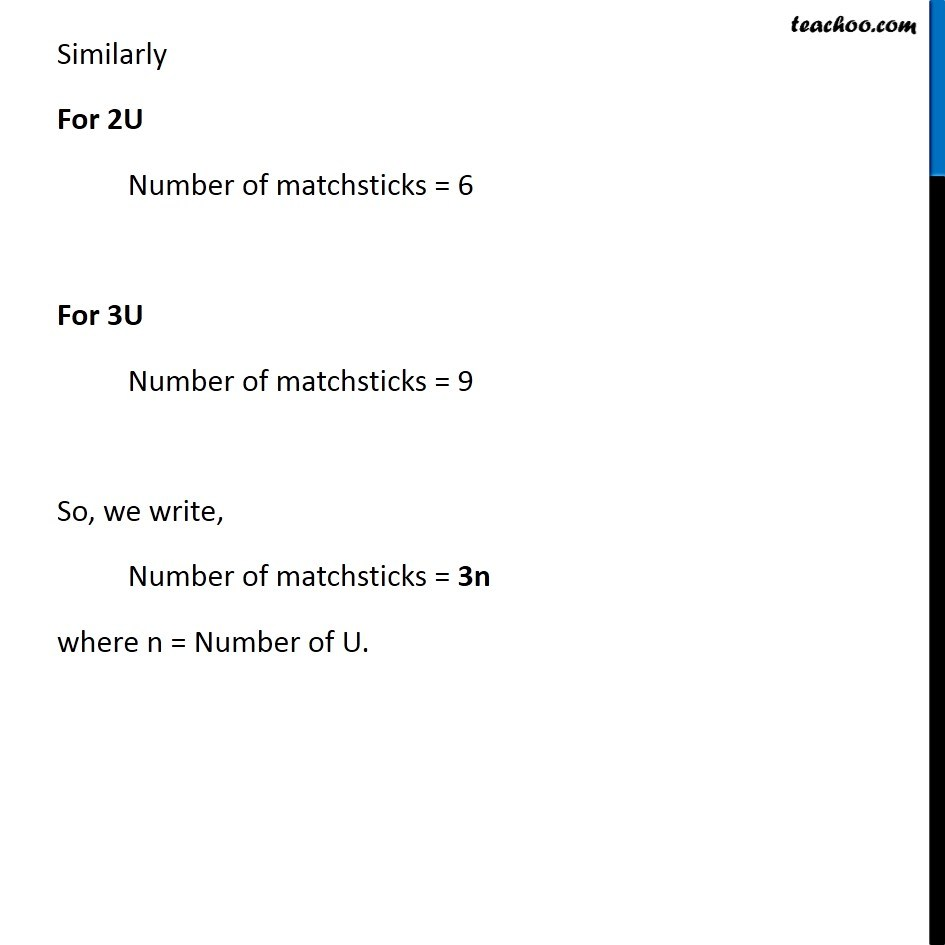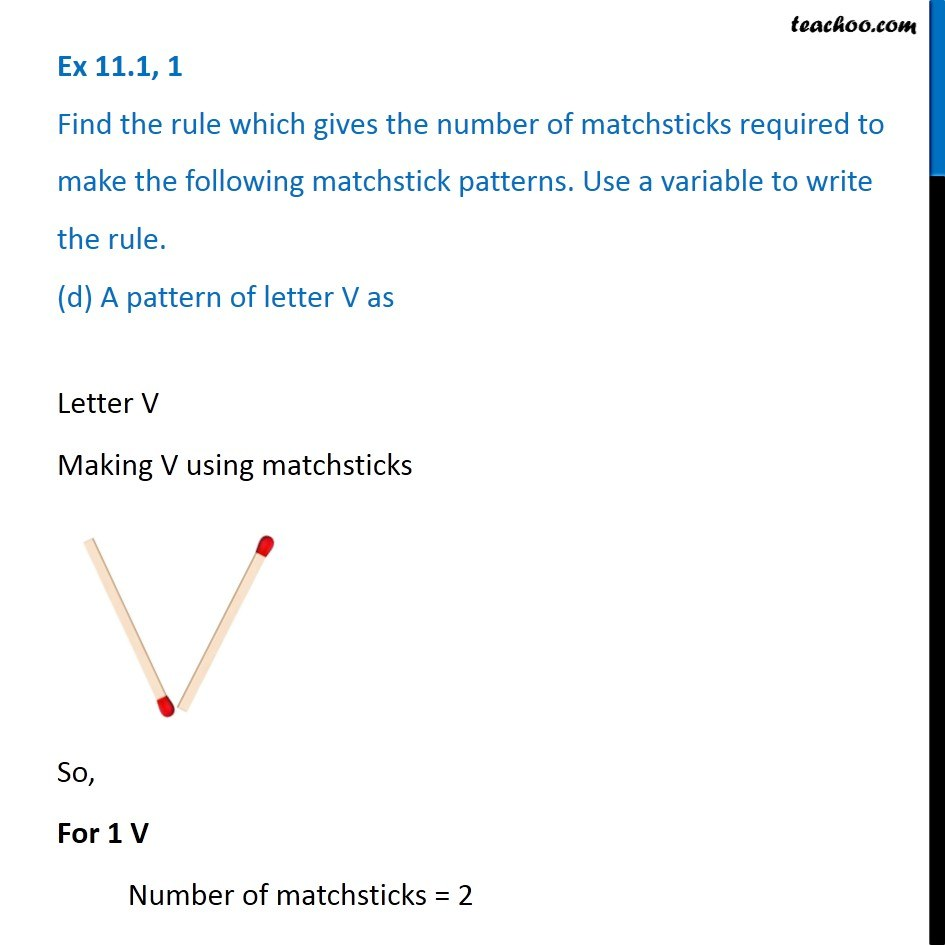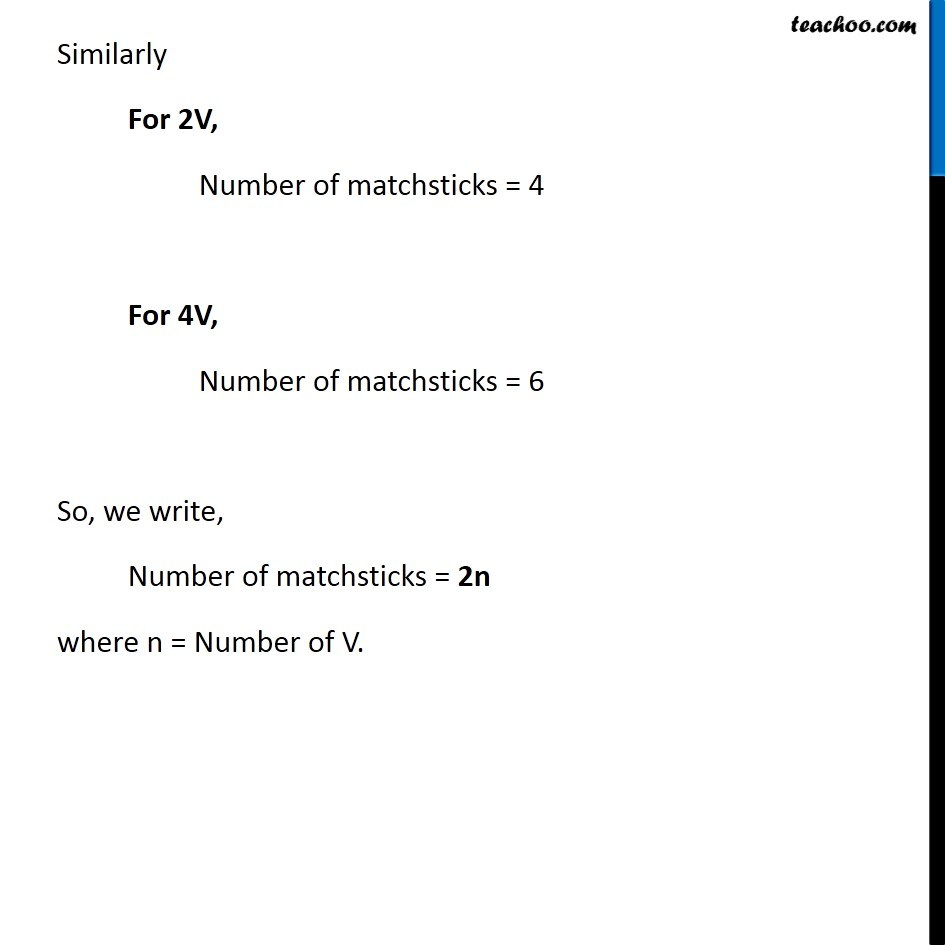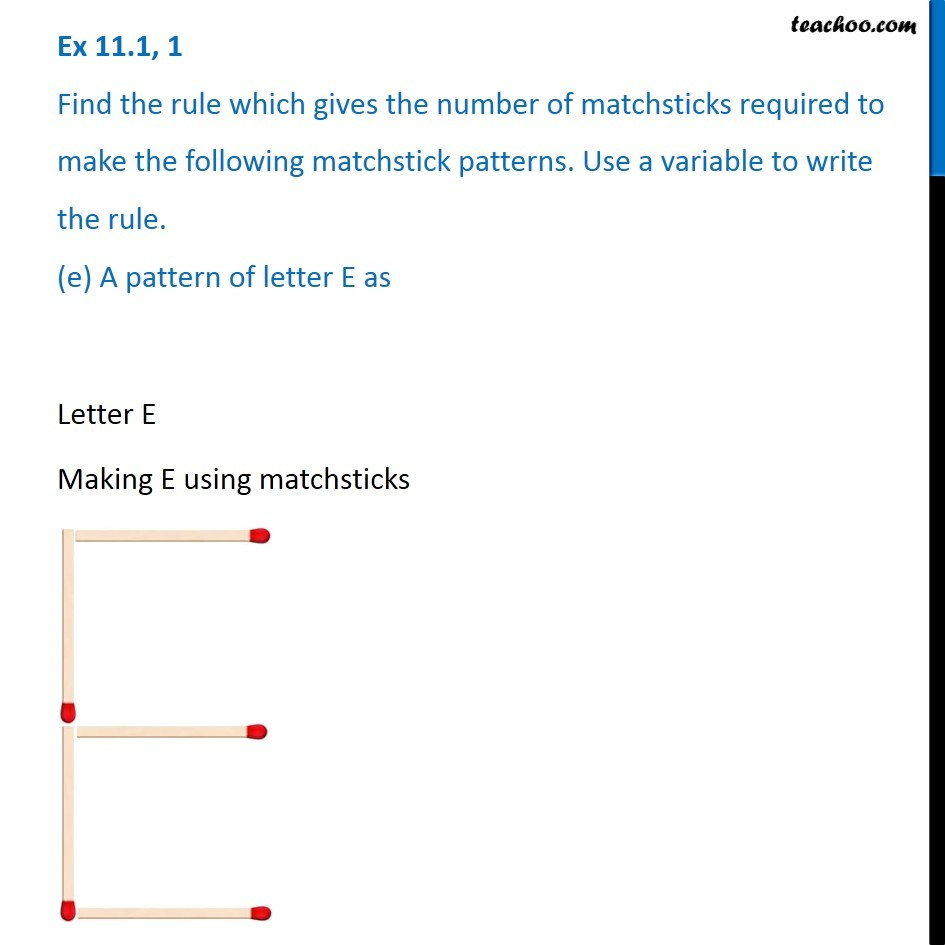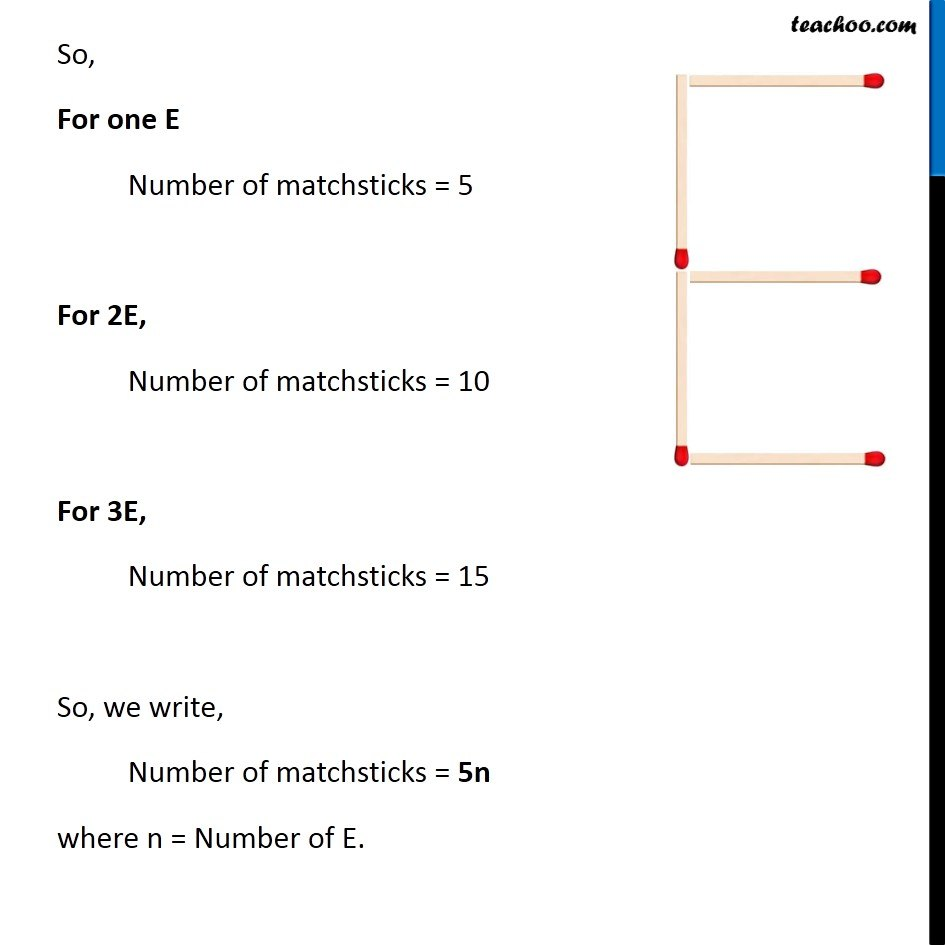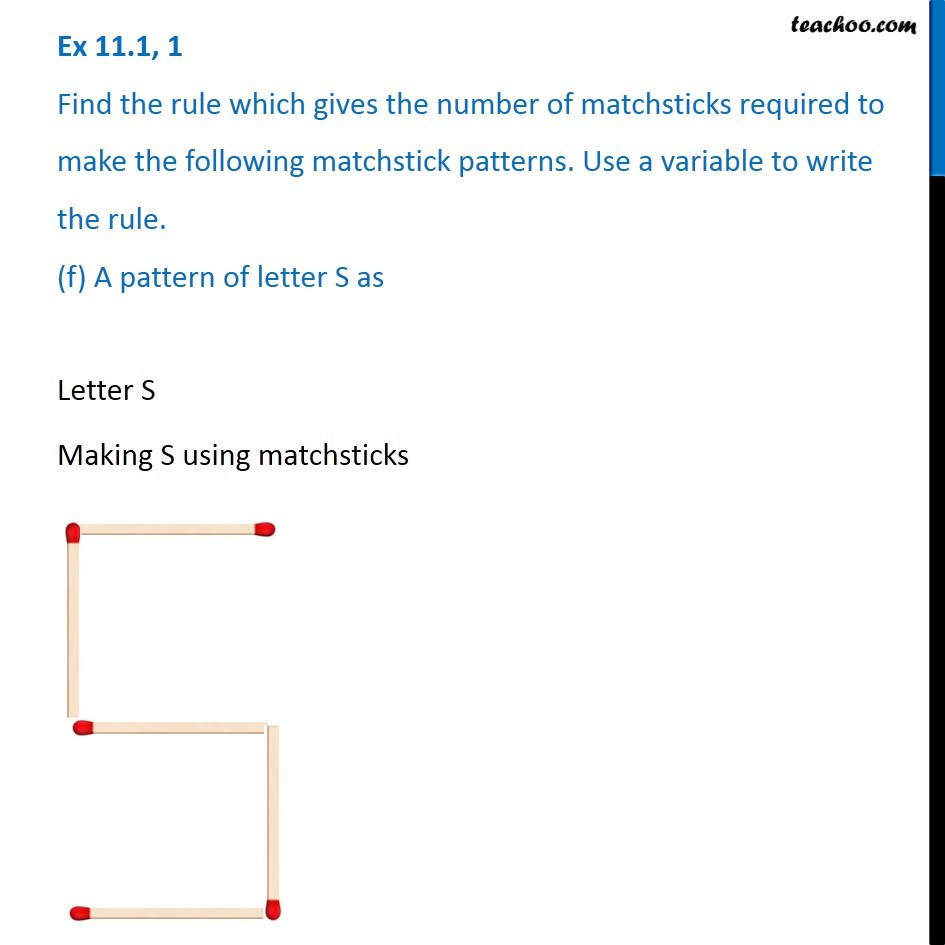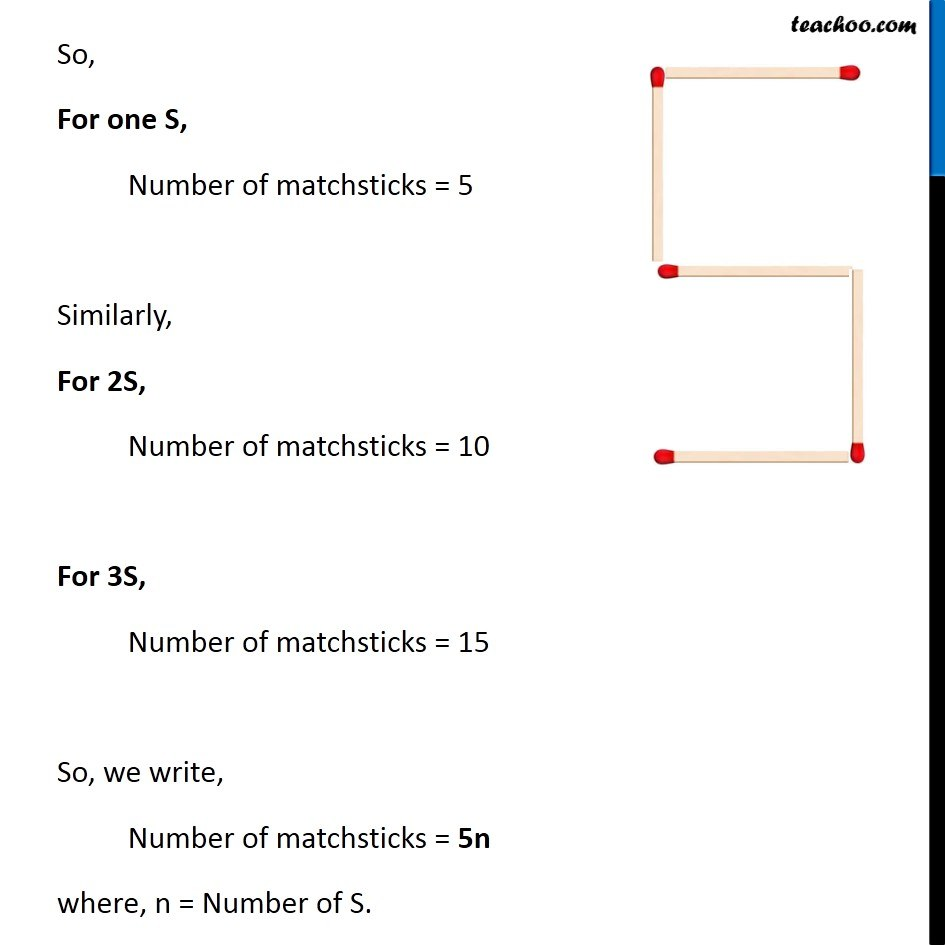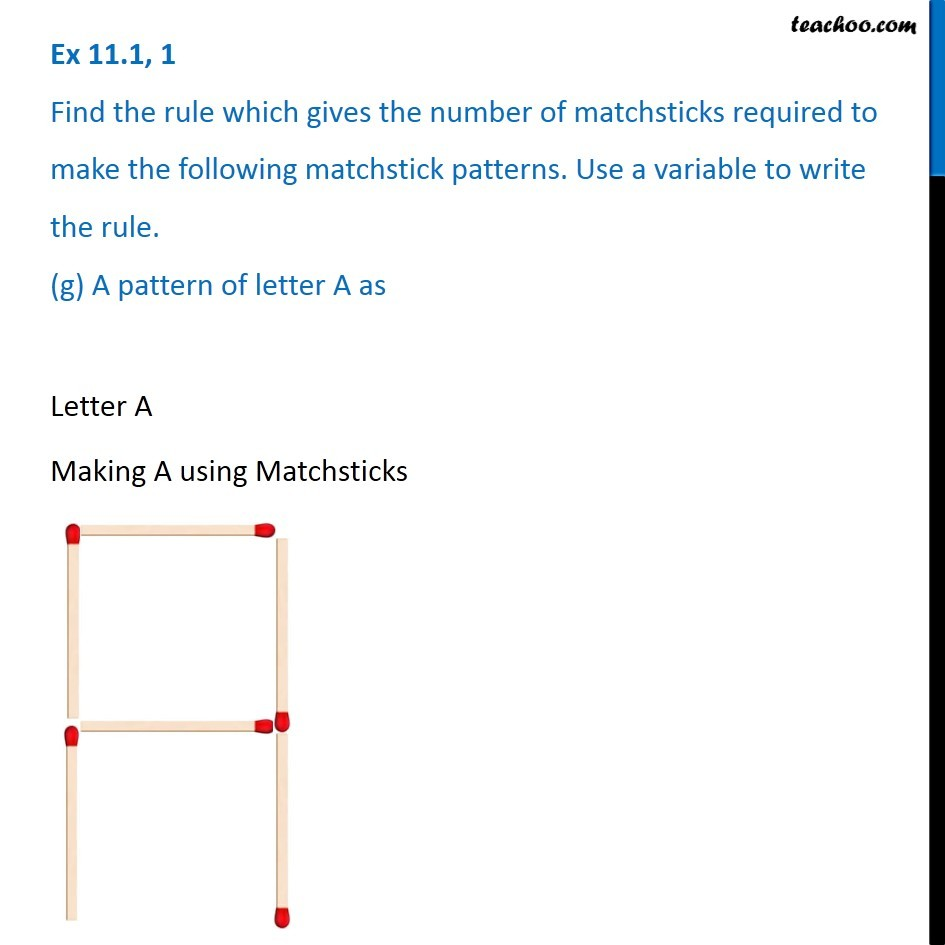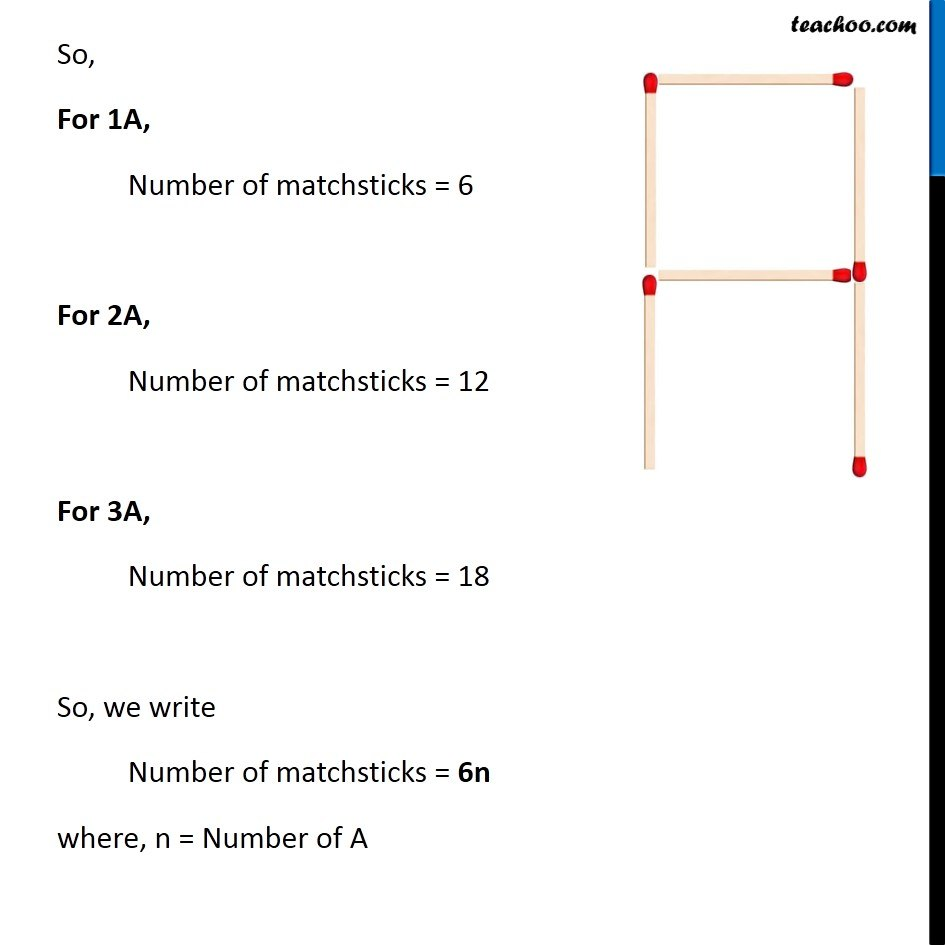Subscribe to our Youtube Channel - https://you.tube/teachoo

1. Chapter 11 Class 6 Algebra
2. Serial order wise
3. Ex 11.1

Transcript

Ex 11.1, 1 Find the rule which gives the number of matchsticks required to make the following matchstick patterns. Use a variable to write the rule. (a) A pattern of letter T as Letter T Making T using Matchsticks So, For IT Number for Matchsticks = 2 For 2T Number for Matchsticks = 4 For 3T Number for Matchsticks = 6 So, we write Number of matchsticks = 2n Where n = Number of T Ex 11.1, 1 Find the rule which gives the number of matchsticks required to make the following matchstick patterns. Use a variable to write the rule. (b) A pattern of letter Z asLetter Z Making Z using matchstick So, For 1Z Number of matchsticks = 3 For 2Z Number of matchsticks = 6 For 3Z Number of matchsticks = 9 So, we write, Number of matchsticks = 3n where n = Number of Z. Ex 11.1, 1 Find the rule which gives the number of matchsticks required to make the following matchstick patterns. Use a variable to write the rule. (c) A pattern of letter U asLetter U Making U using matchsticks So, For 1U Number of matchsticks = 3 Similarly For 2U Number of matchsticks = 6 For 3U Number of matchsticks = 9 So, we write, Number of matchsticks = 3n where n = Number of U. Ex 11.1, 1 Find the rule which gives the number of matchsticks required to make the following matchstick patterns. Use a variable to write the rule. (d) A pattern of letter V asLetter V Making V using matchsticks So, For 1 V Number of matchsticks = 2 Similarly For 2V, Number of matchsticks = 4 For 4V, Number of matchsticks = 6 So, we write, Number of matchsticks = 2n where n = Number of V. Ex 11.1, 1 Find the rule which gives the number of matchsticks required to make the following matchstick patterns. Use a variable to write the rule. (e) A pattern of letter E asLetter E Making E using matchsticks So, For one E Number of matchsticks = 5 For 2E, Number of matchsticks = 10 For 3E, Number of matchsticks = 15 So, we write, Number of matchsticks = 5n where n = Number of E. Ex 11.1, 1 Find the rule which gives the number of matchsticks required to make the following matchstick patterns. Use a variable to write the rule. (f) A pattern of letter S asEx 11.1, 1 Find the rule which gives the number of matchsticks required to make the following matchstick patterns. Use a variable to write the rule. (f) A pattern of letter S asSo, For one S, Number of matchsticks = 5 Similarly, For 2S, Number of matchsticks = 10 For 3S, Number of matchsticks = 15 So, we write, Number of matchsticks = 5n where, n = Number of S. Ex 11.1, 1 Find the rule which gives the number of matchsticks required to make the following matchstick patterns. Use a variable to write the rule. (g) A pattern of letter A as Letter A Making A using Matchsticks So, For 1A, Number of matchsticks = 6 For 2A, Number of matchsticks = 12 For 3A, Number of matchsticks = 18 So, we write Number of matchsticks = 6n where, n = Number of A

Ex 11.1

Chapter 11 Class 6 Algebra
Serial order wise